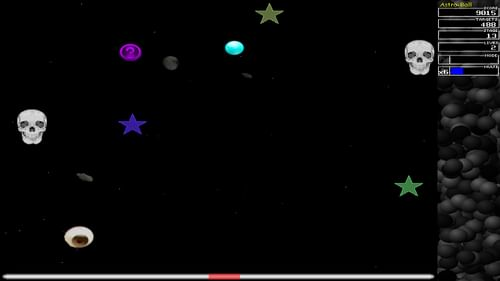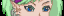-=+=- -=+=- -=+=- -=+=- -=+=- -=+=- -=+=- -=+=- -=+=- -=+=- -=+=- -=+=- -=+=- -=+=- -=+=- -=+=- -=+=- -=+=- -=+=- -=+=- -=+=- -=+=- -=+=- -=+=- -=+=- -=+=- -=+=- -=+=- -=+=- -=+=- (c) WidthPadding Industries 1987 0|177|0 -=+=- -=+=- -=+=- -=+=- -=+=- -=+=- -=+=- -=+=- -=+=- -=+=- -=+=- -=+=- -=+=- -=+=- -=+=- -=+=- -=+=- -=+=- -=+=- -=+=- -=+=- -=+=- -=+=- -=+=- -=+=- -=+=- -=+=- -=+=- -=+=- -=+=-
SoCoder -> Showcase Home -> Arcade

TrickyCreated : 18 October 2019
Edited : 18 October 2019
System : Windows and Mac
Language : Blitz Max

### Astrobounce

Astrobounce

ScreenshotsAstrobounce was a game I developed years ago in BlitzMax, and was built for both Mac and Windows. It was actually the result of a little experiment to see if I could create a bounce effect by using sines. However, pretty soon I made that routine a little more gamey, and poof, the concept for Astrobounce was born.

Originally the game was called Astroball, however since that is a name of game in the Spectrum Z80 classic games, and I didn't want any confusion the game was renamed. I never owned a Z80 so I never played the original Astroball, and I only discovered this later, so my game could not be related in any way. Still the old name could be visible in several parts of the game.The object is easy. All the ball can do is bounce, and you can use the mouse to move it left or right. And well, that's all? Easy? Boring? Think again! As more and more obstacles will get in your way that can destroy you, and in the meantime you must get your 'stars' to score points. There are bonus spheres can can double scores, but also malus spheres that can make stuff be worth nothing. Not to mention the special God Mode, which makes you immortal, float mode, that makes the ball stop bouncing and float in the air, and the crush mode that allows you to destroy your enemies. Can you obtain these? And don't be afraid to share your high score with us.

The game is a class "endless" game, and it's just a matter how long you can survive.

The game is not much (wasn't my intention), but it's still pretty addictive. Check it out

## Latest Update

A very extremely simple, yet addictive game.It was reported that the download was broken. I'll fix that up asap.... In the meantime you can download it from my Google Drive as that download has been confirmed to be workingIt could probably have done with more scoring/dodging mechanics, as it's a teensy bit slow until you reach level 3 or 4. But otherwise it moves along well, and the collisions are nicely done, and there's plenty of swirlyness going on